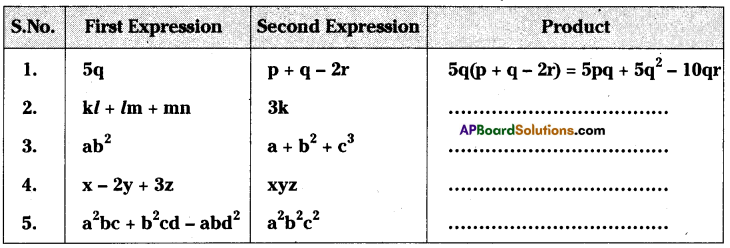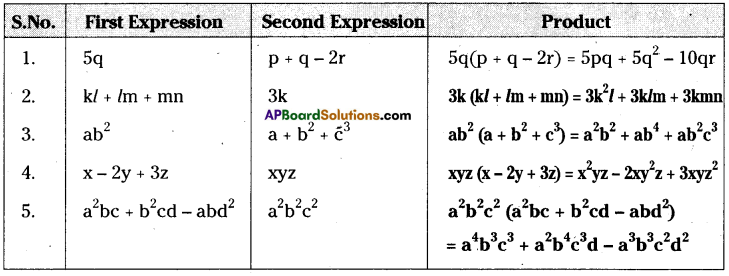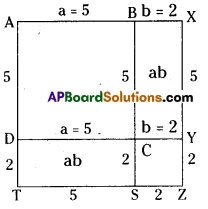# AP Board 8th Class Maths Solutions Chapter 11 Algebraic Expressions Ex 11.2

AP State Syllabus AP Board 8th Class Maths Solutions Chapter 11 Algebraic Expressions Ex 11.2 Textbook Questions and Answers.

## AP State Syllabus 8th Class Maths Solutions 11th Lesson Algebraic Expressions Exercise 11.2Question 1.
Complete the table:Solution:Question 2.
Simplify: 4y(3y + 4)
Solution:
4y(3y + 4) = 4y × 3y + 4y × 4
= 12y2 + 6y

Question 3.
Simplify x(2x2 – 7x + 3) and find the values of it for (i) x = 1 and (ii) x = 0
Solution:
x(2x2 – 7x + 3)
= x × 2x2 – x × 7x + x × 3
= 2x3 – 7x2 + 3x
= 3 × 3 – 1 × 3 – 3 × 1 + 1 × 1
=9 – 3 – 3 + 1 = 4
∴(a – b)2 = 4sq.units
[∵ (3 – 1)2 = 22 = 4](ii)∴ (a – b)2 = a2 – 2ab + b2
Area of ABCD + Area of CYZS
= a2 – 2ab + b2
area of ABCD – area of BXYC – area of DCST + area of CYZS
= 5 × 5 – 2 × 5 – 2 × 5 + 2 × 2
= 25 – 10 – 10 + 4
= 9 sq.units
[∵ (5 – 2)2 = (3)2 = 9]

Question 4.
Add the product: a(a – b), b(b – c), c(c – a)
Solution:
a(a – b) + b(b – c) + c(c – a)
=a × a – a × b + b × b – b × c + c × c – c × a
=a2 – ab + b2 – bc + c2 – ca
=a2 + b2 + c2 – ab – bc – caQuestion 5.
Add the product: x(x + y – r), y(x – y+r), z(x – y – z)
Solution:
x(x + y – r) +y(x – y + r) + z(x – y – z)
= x2 + xy – xr + xy – y2 + yr + zx – yz – z2
= x2 – y2 – z2 + 2xy – xr + yr + zx – yz

Question 6.
Subtract the product of 2x(5x – y) from product of 3x(x+2y)
Solution:
3x(x + 2y) – 2x(5x – y)
=(3x × x + 3x × 2y)-(2x × 5x – 2x × y)
= 3x2 + 6xy – (10x2 – 2xy)
= 3x2 + 6xy- 10x2 + 2xy
= 8xy – 7x2Question 7.
Subtract 3k(5k – l + 3rn) from 6k(2k + 3l – 2rn)
Solution:
6k(2k + 3l – 2m) – 3k(5k – l + 3m)
= 12k2+ 18kl – 12km – 15k2 + 3kl – 9km
= -3k2 + 21kl – 21km

Question 8.
Simplify: a2(a – b + c) + b2(a + b – c) – c2(a – b – c)
Solution:
a2(a – b + c) + b2(a + b – c) – c2(a – b – c)
= a3 – a2b + a2c + ab2 + b3 – b2c – ac2 + bc2 + c3
= a3 + b3 + c3 – a2b + a2c + ab2 – b2c – ac2 – bc2